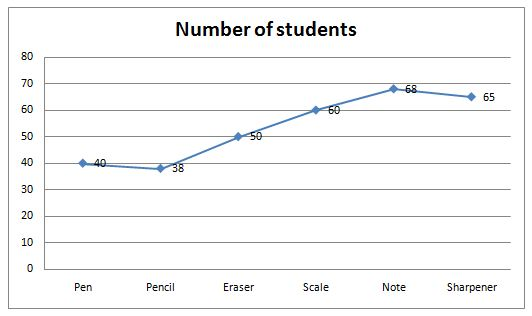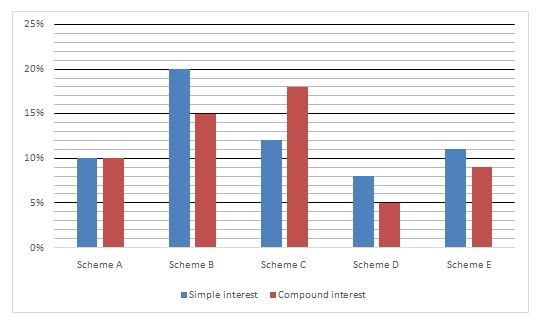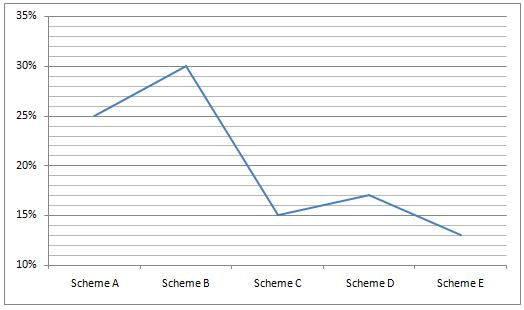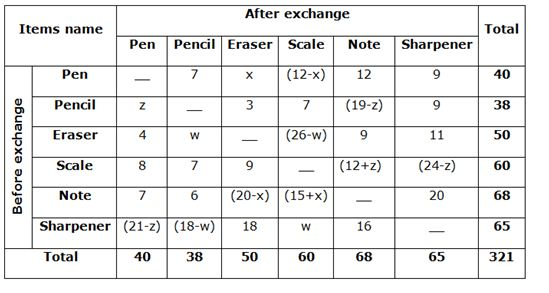# IBPS PO Mains Quantitative Aptitude Questions 2019 – (Day-11)

Dear Aspirants, Our IBPS Guide team is providing new pattern Quantitative Aptitude Questions for IBPS PO Mains 2019 so the aspirants can practice it on a daily basis. These questions are framed by our skilled experts after understanding your needs thoroughly. Aspirants can practice these new series questions daily to familiarize with the exact exam pattern and make your preparation effective.

Check here for IBPS PO Mains Mock Test 2019

[WpProQuiz 7408]

Directions (1 – 5): Read the following information carefully and answer the given questions

In a class of 321 students 321 stationary items are distributed such that every student gets exactly 1 item. There are six different items that are distributed among these students namely Pen, Pencil, Erase, Scale, Note and Sharpener. The following line – graph provides information about the number of students who received Pen, Pencil, Erase, Scale, Note and Sharpener.The students exchanged the items among themselves and after they stopped further exchanging, it was noted that no student had the same item that he/she had earlier. Even after the exchange every students has exactly one item.

Among the students that originally had pen, the number of students now having Pencil, Note and Sharpener is 7, 12 and 9 respectively.

Among the students that originally had Pencil, the numbers of students now having Eraser and scale is 3 and 9 respectively.

Among the students that originally had Eraser, the number of students now having Pen, Note and Sharpener is 4, 9, and 11 respectively.

Among the students that originally had Scale, the number of students now having Pen, Pencil, Eraser is 8, 7 and 9 respectively.

Among the students that originally had Note, the number of students now having Pen, Pencil and Sharpener is 7, 6and 20 respectively.

Among the students that originally had Sharpener, the number of students now having Eraser and Note is 18 and 16 respectively.

1) Among the students that originally had Pen, at least how many students must now have Scale?

a) 5

b) 3

c) 2

d) 1

e) 6

2) If out of the students that originally had Pen, the number of students now having Scale is 8, then find out of the students that now have Eraser?

a) 3

b) 2

c) 4

d) 1

e) 5

3) If among the students that originally had Note, the number of students now having Eraser is 1 more than the number of students now having Scale, then find the difference between the number of the students that now have Sharpener and Scale

a) 4

b) 3

c) 5

d) 6

e) 12

4) If among the students that originally had Sharpener, the number of students now having Eraser is twice the number of students now having Pen, then find out among the students that now having Scale which is one student more than the number of students that now having Pen?

a) 10

b) 9

c) 16

d) 15

e) 18

5) If among the students that originally had Pencil, the number of students now having Note is same as the number of students now having Scale, then find among the students that originally had Sharpener, the number of students now having pen

a) 7

b) 9

c) 8

d) 6

e) 10

Directions (6 – 10): Study the following information carefully and answer the given questions

Given graph shows the percentage of interest in various schemesIn graph percentage of total amount invested by Lalita in different schemesThere was four generations in a family tree Sohan, Sohan father, grandfather, great grandfather. The great grandfather whose age was 85 years in 1862 has 50000 of property with him whom he sold to a zamindar and invested it in the scheme A at simple interest. He died in 1870. After his death, Sohan grandfather received total amount. He invested it in scheme B in the year 1991 at compound interest and died after 2 years on the date of investment. After that Sohan father received Rs. 25 less than the total amount and he invested the amount partly in scheme A at compound interest and partly in the scheme D at simple interest. The ratio of investment done by Sohan father in scheme A to scheme D is 3:4

6) Find the amount received by Sohan if his father dies after three years of investment?

a) Rs.135870

b) Rs.148710

c) Rs.152201

d) Rs.135403

e) None of these

7) Lalita invested Rs.80000 according to the investment plan.  What will be the difference in interest earned if she invests all her money in simple interest for 4 years and compound interest for 2 years following the investment plan?

a) Rs.97104

b) Rs.31480

c) Rs.21379

d) Rs.21889

e) Rs.17699

8) Lalita invested all her money in scheme B at compound interest for 3 years while her sister Babita invested all her money in scheme D at compound interest for 4 years. Total amount received by Lalita is approximately what percent to the total amount received by Babita, if the ratio of amount invested by Lalita to Babita is 5: 3?

a) 209%

b) 220%

c) 231%

d) 198%

e) 239%

9) Find the relation between the following two quantities:

Quantity I: Difference between the interests earned by Lalita on investing in scheme B at simple interest for 3 years and in scheme C at compound interest for 2 years investing Rs. X

Quantity II: Sum of interest earned by Lalita on investing in scheme A and scheme C at simple for 2 years investing Rs. X

a) Quantity I > Quantity II

b) Quantity I < Quantity II

c) Quantity I = Quantity II (or) Relationship cannot be determined

d) Quantity I ≤ Quantity II

e) Quantity I ≥ Quantity II

10) Quantity I: Highest interest earned by Lalita investing at simple interest for 4 years investing Rs.10000

Quantity II: Amount received by Lalita investing in scheme C at compound interest for 2 years investing Rs.10000

a) Quantity I > Quantity II

b) Quantity I < Quantity II

c) Quantity I = Quantity II (or) Relationship cannot be determined

d) Quantity I ≤ Quantity II

e) Quantity I ≥ Quantity II

Directions (1 – 5):From the above table, out of the students that originally had Pen, the number of students now having Scale is ‘12 – x’. Maximum possible value of x is 11.

Therefore minimum possible value of 12 – x

= 12 – 11= 1

From the above table, out of the students that originally had Pen, the number of students now having Scale is 8 = (12-x)

8 = 12 –x

= > x = 4

Out of the students that originally had Pen, the number of students now having Eraser is 4.

Among the students that originally had Note, the number of students now having Scale is a

Among the students that originally had Note, the number of students now having Eraser is b

From the above table, among the students that originally had Note, the total number of students now having Eraser and Scale is

(68 – 35) = 33 = (a + b) — (1)

= > (b – a) = 1 —- (2)

Solve the above equation (1) and (2), we get

= > a = 34/2 = 17

= > b = 16

Required difference = 20 – 17 = 3

Among the students that originally had Sharpener, the number of students now having Eraser is 18

Among the students that originally had Sharpener, the number of students now having Pen be (18/2) = 9

Among the students that originally had Sharpener, the number of students now having Scale = 9 + 1 = 10

From the above table,

Among the students that originally had Pencil, the number of students now having Note = among the students that originally had Pencil, the number of students now having Scale = 7

Among the students that originally had Pencil, the number of students now having Pen = 38 – (3+7+9+7) = 38 – 26 = 12 = z

Among the students that originally had Sharpener, the number of students now having pen = (21 – z)

= 21 – 12 = 9

Directions (6 – 10):

For Great Grand Father

Principal Amount=Rs.50,000

Simple interest = p*r*t/100

=50000*10*8/100

=Rs.40,000

Amount= Principal + Simple Interest

=50000 + 40000 => Rs 90,000

For Grand Father

Principal amount = Rs.90000

Scheme B compound interest =15%

Time=2 years

Amount on compound interest = p*(1+r/100)t

=90000*(1+15/100)2

=>119025

For Sohan Father

Amount received by Sohan Father = 119025 – 25 = 119000

Amount invested in scheme A at compound interest for 3 years=119000*3/7

=>Rs.51000

Amount on compound interest =p*(1+r/100)t

=51000*(1+10/100)3

=>Rs.67881

Amount invested in scheme D at simple interest for 3 years=119000*4/7

=>Rs.68000

Amount on simple interest= P+ (P*r*t/100)

= 68000 + (68000*8*3/100) = 68000 + 16320

=>Rs.84320

Amount Sohan Received = 67881+ 84320=>Rs.152201

For Scheme A:

Amount invested=25% of 80000= Rs.20000

Interest earned through simple interest = p*r*t/100

=20000*10*4/100 = Rs.8000

Interest earned through compound interest = = p*(1+r/100)t – P

= 20000*(1+10/100)2 – 20000

Interest earned=24200 – 20000 = 4200

Difference of interest= 8000 – 4200 = Rs.3800

For Scheme B:

Amount invested = 30% of 80000 = Rs.24000

Interest earned through simple interest = p*r*t/100

= 24000*4*20/100 = Rs.19200

Interest earned through Compound Interest= p*(1+r/100)t – p

= 24000*(1+15/100)2 – 24000

= Rs.31740 – 24000 = Rs.7740

Difference of interest = 19200 – 7740 = Rs.11460

For Scheme C:

Amount invested = 15% of 80000 =Rs.12000

Interest earned through simple interest=p*r*t/100

=12000*12*4/100 = Rs. 5760

Interest earned through Compound Interest= p*(1+r/100)t – p

=12000*(1 + 18/100)2 – 12000

=12000*59/50*59/50 – 12000

=16709 – 12000 = Rs.4709

Difference of interest= 5760 – 4709 = Rs.1051

For Scheme D:

Amount invested = 17% of 80000

Amount invested = Rs.13600

Interest earned through simple interest=p*r*t/100

=13600*8*4/100 =Rs.4352

Interest earned through Compound Interest= p*(1+r/100)t – p

= 13600*(1+5/100)2 – 13600

= 13600*105/100*105/100 – 13600

= 14994 – 13600 = Rs.1394

Difference of interest= 4352 – 1394= Rs.2958

For Scheme E:

Amount invested = 13% of 80000 = 10400

Interest earned through simple interest=p*r*t/100

=10400*11*4/100

=Rs.4576

Interest earned through Compound Interest= p*(1+r/100)t – p

=10400* (1+ 9/100)2 – 10400

= 10400*109/100*109/100 – 10400

=12356 – 10400 = 1956

Difference of interest = 4576 – 1956 = > Rs.2620

Total difference = 2620 + 2958 + 1051 + 3800 + 11460

= Rs.21889

Let the amount invested by Lalita is 5X

Let the amount invested by Babita is 3X

Amount received by Lalita = p*(1+r/100)t

=5X*(1 +15/100)3

=5X*115/100*115/100*115/100 = 7.60 X

=3X*(1 +5/100)4

=3X*105/100*105/100*105/100*105/100

=3.64X

Required percentage= 7.60X/3.64X * 100

= 208.79% = 209%

Quantity I:

For Scheme B

Amount invested = X*30% = Rs.0.3X

Interest earned through simple interest=p*r*t/100

0.3X*20*3/100 = 0.18X

For Scheme C

Amount invested= x*15% = 0.15X

Interest earned through compound interest= p*(1+r/100)t – p

= 0.15X*(1+18/100)2 – 0.15X

= 0.208X – 0.15X = 0.058X

Difference = 0.18X – 0.058X = 0.122X

Quantity II:

For Scheme A

Amount invested=X*25% = Rs.0.25X

Interest earned through simple interest=p*r*t/100

=0.25X*10*2/100 = 0.05X

For Scheme C

Amount invested=X*15% = Rs.0.15X

Interest earned through simple interest=p*r*t/100

=0.15X*12*2/100 = 0.036X

Total interest = 0.05X+0.036X

=>0.086X

Quantity I < Quantity II

Quantity I: Amount invested=10000

For scheme A

Amount invested=10000*25% = Rs.2500

Interest earned through simple interest=p*r*t/100

=2500*10*4/100 = 1000

For Scheme B

Amount invested=10000*30% = Rs.3000

Interest earned through simple interest=p*r*t/100

=3000*20*4/100 = 2400

For Scheme C

Amount invested=10000*15% = Rs.1500

Interest earned through simple interest=p*r*t/100

=1500*12*4/100 = 720

For Scheme D

Amount invested=10000*17% = Rs.1700

Interest earned through simple interest=p*r*t/100

=1700*8*4/100 = 544

For Scheme E

Amount invested=10000*13% = Rs.1300

Interest earned through simple interest=p*r*t/100

=1300*11*4/100 = 572

Highest interest is earned by scheme B

(Or)

Amount invested in scheme B is highest at the same time Rate of interest in scheme B is highest compare than other schemes.

Amount invested=10000*30% = Rs.3000

Interest earned through simple interest=p*r*t/100

=3000*20*4/100 = 2400

Quantity I = 2400

Quantity II:

Amount invested=10000*15% = Rs.1500

Interest earned through compound interest= p*(1+r/100)t

=1500*(1+18/100)2

=1500*118/100*118/100

=Rs.2088

Quantity II = 2088

Hence Quantity I > Quantity II